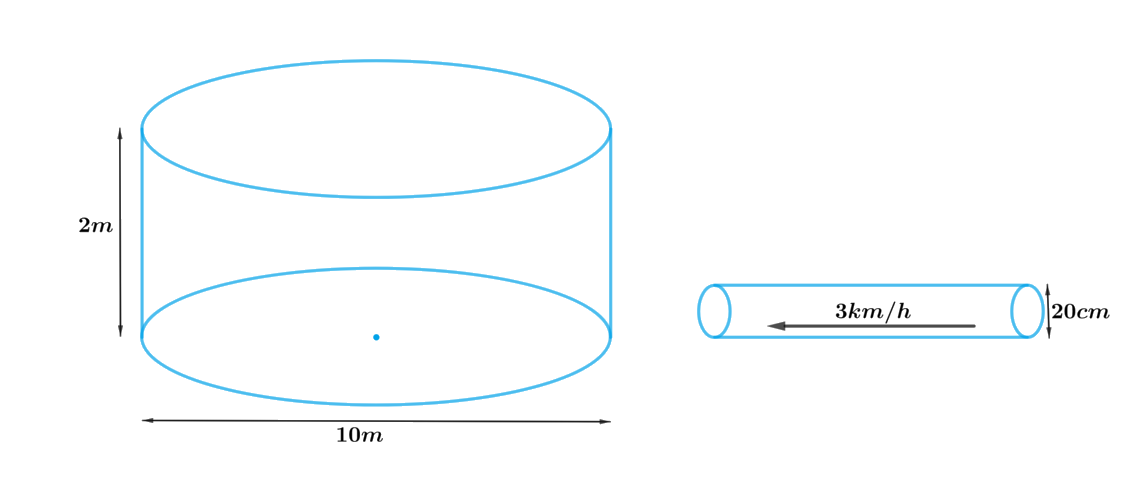# Ex.13.3 Q9 Surface Areas and Volumes Solution - NCERT Maths Class 10

Go back to  'Ex.13.3'

## Question

A farmer connects a pipe of internal diameter $$20\,\rm{cm}$$ from a canal into a cylindrical tank in her field, which is $$10\,\rm{m}$$ in diameter and $$2\,\rm{m}$$ deep. If water flows through the pipe at the rate of $$3\,\rm{km/h}$$, in how much time will the tank be filled?

## Text Solution

What is known?

Internal diameter of the pipe is $$20\,\rm{cm}$$ and speed of water flows through the pipe is $$3\,\rm{km/h.}$$

Diameter of the cylindrical tank is $$10\rm{m}$$ and depth is $$2\,\rm{m}$$

What is unknown?

Time taken to fill the tank.

Reasoning:

Draw a figure to visualize the shapes betterFrom the figure it’s clear that shape of the cross-section of the pipe is cylindrical. So, volume of the water, flowing at the speed of $$3\,\rm{km/h}$$ through the pipe to fill the tank will be same as the volume of water in the cylindrical tank.

To find the volume of the water we need to find length of the water flowing through the pipe at the speed of $$3\,\rm{km/h}$$

Hence, length of the water flowing through the pipe in $$1\rm{hour(60minutes)} = 3\rm{km}$$

Length of the water flowing through the pipe in $$1\rm{minute} = 50\rm{m}$$

We will find the volume of the water by using formula;

Volume of the cylinder $$= \pi {r^2}h$$

where $$r$$ and $$h$$ are radius and height of the cylinder respectively.

Therefore,

Volume of water flowing through the pipe $$=$$ volume of water in the cylindrical tank

Steps:

Radius of the cylindrical tank,\begin{align} R = \frac{{10 \rm m}}{2} = 5 \rm m\end{align}

Depth of the cylindrical tank,$$H = 2 \rm m$$

Radius of the cylindrical pipe,\begin{align} r = \frac{{20 \rm cm}}{2} = \frac{{10}}{{100}} \rm m = 0.1m\end{align}

Length of the water flowing through the pipe in $$1\rm{hour (60minutes)} = 3\rm{km}$$

Length of the water flowing through the pipe in $$1\rm{minute,}$$\begin{align} h = \frac{{3 \rm km}}{{60}} = \frac{{3 \times 1000 \rm m}}{{60}} = 50 \rm m\end{align}

Let the tank be filled completely in $$t$$ minutes.

Volume of water flowing through pipe in $$t$$ minutes $$=$$ volume of water in cylindrical tank

\begin{align}t \times \pi {r^2}h &= \pi {R^2}H\\t &= \frac{{{R^2}H}}{{{r^2}h}}\\&= \frac{{5 \rm m \times 5 \rm m \times 2 \rm m}}{{0.1 \rm m \times 0.1 \rm m \times 50 \rm m}}\\&= 100\end{align}

Therefore, the cylindrical tank will be filled in $$100$$ minutes.

Learn from the best math teachers and top your exams

• Live one on one classroom and doubt clearing
• Practice worksheets in and after class for conceptual clarity
• Personalized curriculum to keep up with school